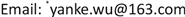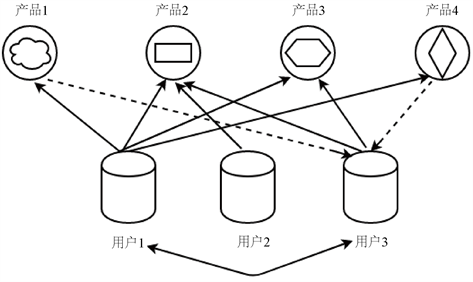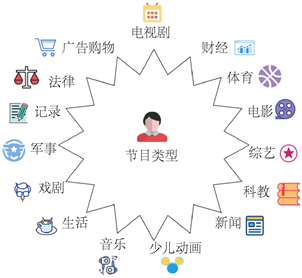﻿ 基于协同过滤算法的电视用户个性化推荐 Personalized Recommendation of TV Users Based on Collaborative Filtering Algorithm

Statistics and Application
Vol. 08  No. 04 ( 2019 ), Article ID: 31659 , 9 pages
10.12677/SA.2019.84071

Personalized Recommendation of TV Users Based on Collaborative Filtering Algorithm

Xingxing Chen, Ruitao Li, Junhua Liao, Yanke Wu*

School of Mathematics and Computer Science, Guangdong Ocean University, Zhanjiang GuangdongReceived: Jul. 19th, 2019; accepted: Aug. 1st, 2019; published: Aug. 12th, 2019ABSTRACT

In order to integrate and utilize existing data better, and improve the marketing effectiveness of TV program products, this paper processes the data of the watching information of TV users and establishes the user preference model to obtain three preference types of each user, and then uses the collaborative filtering algorithm to carry out personalized recommendation of individual users. In addition, we use K-means algorithm as well as the KNN algorithm to divide the users into groups and obtain the recommendation of each user group, thereby effectively solving the problem of personalized recommendation of the users.

Keywords:K-means, KNN, Collaborative Filtering, Personalized Recommendation1. 引言

2. 协同过滤算法Figure 1. Classification of television programs

1) 建立用户模型

2) 寻找目标用户的邻居

$Sim\left(i,j\right)=\frac{{\sum }_{q=1}^{15}\left({R}_{i,q}-\stackrel{¯}{{R}_{i}}\right)\left({R}_{j,q}-\stackrel{¯}{{R}_{j}}\right)}{\sqrt{{\sum }_{q=1}^{15}\left({R}_{i,q}-\stackrel{¯}{{R}_{i}}\right)\stackrel{¯}{{R}_{j}}}\sqrt{{\sum }_{q=1}^{15}{\left({R}_{j,q}-\stackrel{¯}{{R}_{j}}\right)}^{2}}}$ (1)

3) 产生目标用户的推荐产品

${P}_{i,q}=\stackrel{¯}{{R}_{i}}+\frac{{\sum }_{j\in U}sim\left(i,j\right)×\left({R}_{j,q}-\stackrel{¯}{{R}_{j}}\right)}{{\sum }_{j\in U}\left(|sim\left(i,j\right)|\right)}$ (2)

3. 数据来源及预处理

1) 去除回看信息重复和用户点播信息重复的数据、观看时间为空的记录和观看时长小于一分钟的记录。

2) 将其中冗余的属性以及与挖掘过程不相关的属性剔除得到处理后的数据，如表1所示。

4. 建立用户偏好模型

4.1. 构建用户偏好类型Figure 2. The type of TV program

4.2. 用户类型的偏好度

$interes{t}_{\left(i,q\right)}=\frac{{\sum }_{k=1}^{n}\text{\hspace{0.17em}}{t}_{label\left(i,q\right)}\left[k\right]}{{\sum }_{q=1}^{m}{\sum }_{k=1}^{n}\text{\hspace{0.17em}}{t}_{label\left(i,q\right)}\left[k\right]}$ (3)

$Loyalt{y}_{\left(i,q\right)}=\frac{{\sum }_{k=1}^{n}\text{\hspace{0.17em}}{t}_{label\left(i,q\right)}\left[k\right]}{{\sum }_{k=1}^{n}\text{\hspace{0.17em}}{t}_{program\left(i,q\right)}\left[k\right]}$ (4)

$Labe{l}_{\left(i,q\right)}={\omega }_{1}Loyalt{y}_{\left(i,q\right)}+{\omega }_{2}interes{t}_{\left(i,q\right)}$ (5)

5. 协同过滤算法对单个用户的推荐

5.1. 建立用户模型

5.2. 寻找目标用户的邻居

5.3. 产生目标用户的推荐类型

5.4. 实现用户的节目推荐

6. 用户的打包推荐

6.1. K-means聚类分析与KNN算法进行用户分群

6.2. 节目产品的打包和推送Table 8. Recommended program package for each user group

7. 结论及展望

1) 各个电视台的节目类型时间比例是根据各个电视台(分周一至周五和周末)的常规播放规律来进行计算的，与准确的节目类型播放规律会有一定的误差。

2) 评分较高的节目推荐给目标用户，往往会导致推送的不精准和有一大部分评分较低的节目没有用户去收看。

Personalized Recommendation of TV Users Based on Collaborative Filtering Algorithm[J]. 统计学与应用, 2019, 08(04): 522-530. https://doi.org/10.12677/SA.2019.84071

1. 1. 周虹君, 殷复莲, 陈怡婷, 周嘉琪, 伊成昱. Spark框架下的受众分群及矩阵分解的推荐算法研究[J]. 互联网 + 健康, 2016, 20(2): 139-141.

2. 2. 张晓阳. 基于受众收视行为分析的电视节目编排策略刍探[J]. 企业家天地, 2011, 3(1): 187-188.

3. 3. 高肖俊, 丁云强. 基于用户收视兴趣模型的广电客户分群及精确化营销系统建设[J]. 视听界, 2016, 3(2): 1-3.

4. 4. 夏欢. 基于组合策略的IPTV节目推荐[D]: [硕士学位论文]. 昆明: 云南大学, 2016.

5. 5. 沈建军. 面向互动电视的影视节目推进系统研究与实现[D]: [硕士学位论文]. 杭州: 复旦大学, 2012.

6. 6. 钟智, 朱曼龙, 张晨, 黄樑昌. 最近邻分类方法的研究[J]. 广西师范学院, 2011, 5(1): 2-3.

7. 7. 赵营. 基于协同过滤算法的习题推荐[J]. 科技经济导刊, 2016, 6(5): 136.

8. 8. 李楚桐, 莫赞. 基于协同过滤算法的推荐系统研究[J]. 信息通信, 2018, 1(2): 38-39.

9. 9. 王国霞, 刘贺平. 个性化推荐系统综述[J]. 计算机工程与应用, 2012, 48(7): 66-67.

10. 10. 于玉龙, 王秀芳. 改进的协同过滤推荐算法[J]. 互联网 + 通信, 2016, 1(1): 12.

11. 11. 张岩. 基于协同过滤的个性推荐算法研究及系统实现[D]: [硕士学位论文]. 北京: 北京化工大学, 2014.

12. NOTES

*通讯作者。